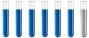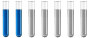## Enthalpy of reaction using one equation and its enthalpy

Chemistry and homework help forum.

Organic Chemistry, Analytical Chemistry, Biochemistry, Physical Chemistry, Computational Chemistry, Theoretical Chemistry, High School Chemistry, Colledge Chemistry and University Chemistry Forum.

Share your chemistry ideas, discuss chemical problems, ask for help with scientific chemistry questions, inspire others by your chemistry vision!

Please feel free to start a scientific chemistry discussion here!

Discuss chemistry homework problems with experts!

Ask for help with chemical questions and help others with your chemistry knowledge!

Moderators: expert, ChenBeier, Xen

Dhamnekar Winod
Sr. Staff MemberPosts: 192
Joined: Sat Nov 21, 2020 10:14 am
Location: Mumbai[Bombay],Maharashtra State,India

### Enthalpy of reaction using one equation and its enthalpy

How much heat is produced when 100 mL of 0.250 M HCl (density, 1.00 g/mL) and 200 mL of 0.150 M NaOH (density, 1.00 g/mL) are mixed?
HCl(aq) + NaOH(aq) → NaCl(aq) + H₂O(l) ∆H°₂₅°c = −58 kJ If both solutions are at the same temperature and the heat capacity of the products is 4.19 J/g℃, how much will the temperature increase? What assumption did you make in your calculation?

My answer: Reaction given produces 58 kJ of heat with 1 mol of NaOH. We have 0.150 M of NaOH (limiting reagent) so it will produce 0.150 M × -58 kJ/1.0 M= -8.7 kJ of heat.
We know that q=cm∆T, substituting the values we have , in the equation -8.7 kJ = 4.19 J/g℃ × 300 g × ∆T, ⇒ ∆T= 6.9℃

Last edited by Dhamnekar Winod on Sat Jul 03, 2021 9:44 am, edited 1 time in total.
Any science consists of the following process. 1) See 2)Hear 3)Smell,if needed 4)Taste, if needed 5)Think 6)Understand 7)Inference 8)Take decision [Believe or disbelieve, useful or useless, true or false, cause or effect, any other criteria]
Orcio_Dojek
Jr. MemberPosts: 7
Joined: Sun Jun 20, 2021 7:55 am

### Re: Enthalpy of reaction using one equation and its enthalpy

How much heat is produced when 100 mL of 0.250 M HCl (density, 1.00 g/mL) and 200 mL of 0.150 M NaOH (density, 1.00 g/mL) are mixed?
HCl(aq) + NaOH(aq) → NaCl(aq) + H₂O(l) ∆H°₂₅°c = −58 kJ If both solutions are at the same temperature and the heat capacity of the products is 4.19 J/g℃, how much will the temperature increase? What assumption did you make in your calculation?

My answer: Reaction given produces 58 kJ of heat with 1 mol of NaOH. We have 0.150 M of NaOH (limiting reagent) so it will produce 0.150 M × -58 kJ/1.0 M= -8.7 kJ of heat.
We know that q=cm∆T, substituting the values we have , in the equation -8.7 kJ = 4.19 J/g℃ × 300 g × ∆T, ⇒ ∆T= 6.92℃